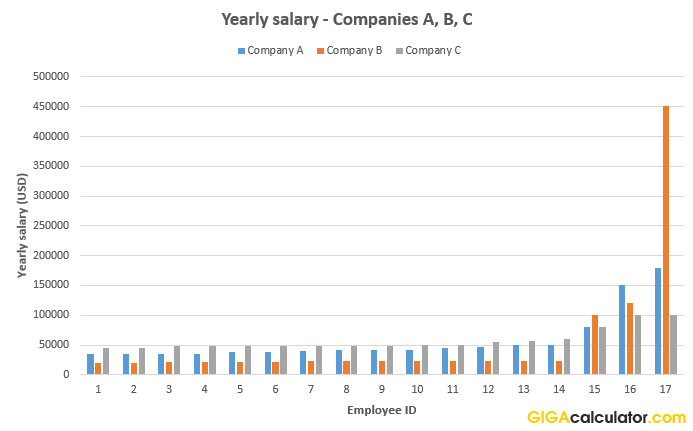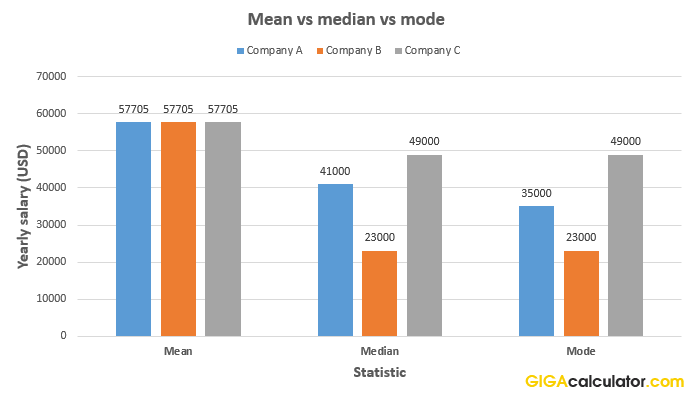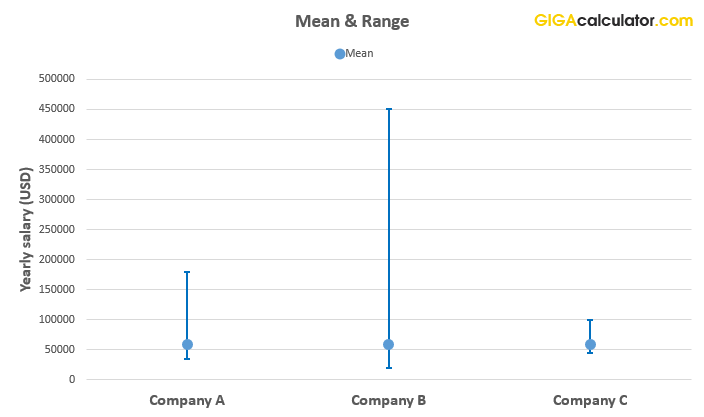# Mean, Median & Mode Calculator

Use this calculator to easily calculate the arithmetic mean, median, mode, and range of a set of numbers. Find the mean, median, and mode of any data set.

Share calculator:

Embed this tool:
get code

## Mean, median, mode, and range

These are the most popular summarizing statistics used to describe a data set using one or several numbers. The mean, median and mode can all be called an "average" in certain literature, but using their proper technical names is recommended to avoid confusion. Adding "arithmetic" also helps, since there is a geometric mean, a harmonic mean, a geometric median, etc. and it is not always immediately clear from context which one you are referring to.

In common English "average" and even "mean" can be used as synonyms to "typical", which can be highly misleading in many situations where the distribution is skewed or dispersed (see the example below). In our mean, median, mode & range calculator we are just calling them by their short name.

Here are short definitions and when each is most appropriate, and examples based on the set of numbers: 1, 2, 3, 3, 5, 10, 18, 20, 28

### The arithmetic mean

The arithmetic mean is the sum of all values, divided by their count. The mean of the above set, as calculated by our Mean, Median & Mode calculator, is 10. Summing the differences between the mean and each element of a set results in zero, so the mean is an unbiased statistic. It also minimizes the root mean squared error (RMSE) and is in this sense the single best predictor of the set.

You should use the mean when you want to present a value which, if you were to use it to guess about any value of the set, you would be least far from each element of the set, on average. The mean is easily understood, but just so often misunderstood, especially when used with skewed distributions or distributions with a big range.

### The median

The median is the value that splits an ordered set of data in half so a summary statistic which minimizes the average distance between itself and each number. The median of the above set, which is ordered from lowest to highest, is 5. Half of the elements would have a value smaller than the median, and half of them will have a value larger than the median. The median for a set with an even number of elements is usually calculated by taking the arithmetic mean of the two elements around the mid-point.

The median is useful when we want to speak about halves, about a reference point from which 50% of the values fall above or below. It is thus used in income and revenue statistics, sometimes in height and weight statistics - for example in CDC data on people 0-21 years old.

### The mode

The mode is most frequently occurring value in a set. It has the property of minimizing the number of times a number from a set is not equal to that set's mode. The mode of the example set is 3, as it occurs twice, and every other value - only once. A set can have more than one modes - it can be bimodal or even multimodal. For example, adding "20" to the above set, would result in it having two modes: 3 and 20.

The mode is used in elections and different kinds of votes, where the counting is essentially the operation of determining the mode of the votes cast. A mode is also your best bet, if your goal is to guess the exact value of a randomly drawn element from the set. You can certainly use the above mode calculator to order the values by their frequency and show the mode (or modes) of the set of interest.

### The range

The range is simply the lowest and highest value in a set. If the set is ordered, they are immediately obvious, otherwise you need to order the set in ascending order and then take the first and last value. In the above example set, the range is 1-28.

The range is the appropriate statistic to calculate using our range calculator above when you want to know the minimum and maximum possible value, values under which and over which there are no other values. These are often extreme cases in population statistics, disease symptoms, and others.

## Mean vs median vs mode vs range: a practical example

Let us review fictitious yearly salary data for three companies: A, B, and C, all with 17 employees. The raw data looks like so:Let us now see each of the three statistics (mean, median, and mode), compared for the three companies:We can see that the arithmetic average is exactly the same for the three (\$57,705.88), the median varies significantly (from \$23,000 to \$49,000), and so does the mode. For two of the companies the median is equal to the mode. In many cases, we would find the median to be the most desirable statistic based on such considerations, but ultimately it is the question at hand that should inform which one to use. See When to Use Mean, Median, or Mode for a deeper discussion.

Here is the same data summarized by its arithmetic mean and the min-max range:Having the range is quite informative about the distribution of salaries within the companies, and having the average as well gives as an idea of where the density of the distribution falls. (calculated using our mean and range calculator)

The above is just one example. There are many examples of data coming from totally different distributions that might have the same summary statistics, so, when possible, always request the raw data or at least a plot of the whole distribution of the data, accompanied by the statistics thought to be most informative.

#### Cite this calculator & page

If you'd like to cite this online calculator resource and information as provided on the page, you can use the following citation:
Georgiev G.Z., "Mean, Median, Mode Calculator", [online] Available at: https://www.gigacalculator.com/calculators/mean-median-mode.php URL [Accessed Date: 28 Sep, 2023].